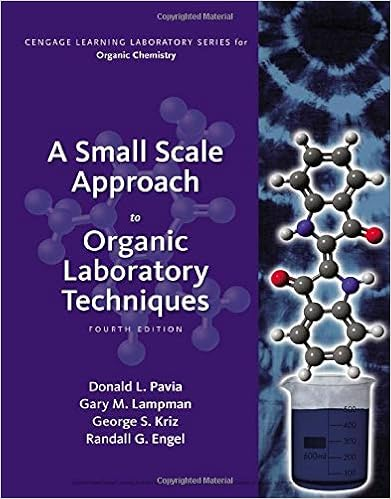# Introduction in this lab we will be able to use our

• Lab Report
• 6

This preview shows page 3 - 6 out of 6 pages.

##### We have textbook solutions for you!
The document you are viewing contains questions related to this textbook.The document you are viewing contains questions related to this textbook.
Chapter T19 / Exercise 5
A Small Scale Approach to Organic Laboratory Techniques
Lampman/PaviaExpert Verified
Introduction In this lab we will be able to use our results from last week’s lab to find the percentage oxalate ion in the Potassium Ferric Oxalate Trihydrate. To do this we will use titration, a type of volumetric analysis. To find the percentage oxalate ion using titration we will have to add a Potassium Permanganate solution, whose concentration is previously known, to a solution of the Oxalate until the reaction occurs. Experimental Data Standardization of the Permanganate Solution Initial Volumes of KMnO 4 : Trial One: 49.02mL Trial Two: 49.5 mL Trial Three: 50.0mL Volumes of KMnO 4 Used for Titration: Trial One: 38.69mL Trial Two: 36.61mL Trial Three: 38.02mL Na 2 C 2 O 4 Masses (Fine white powder): Trial One: .1106g Trial Two: .1096g Trial Three: .1106g 6M H 2 SO 4 (Clear liquid) - 5mL After the Titration the Solution turned a pale rosy pink. Mass of Na 2 C 2 O 4 : 133.92g (2 x 22.98)(12 x 2)(16 x 4) = 133.92g Moles of Na 2 C 2 O 4 : Trial One: 8.25 x 10 -4 mol of NaC 2 O 4
##### We have textbook solutions for you!
The document you are viewing contains questions related to this textbook.The document you are viewing contains questions related to this textbook.
Chapter T19 / Exercise 5
A Small Scale Approach to Organic Laboratory Techniques
Lampman/PaviaExpert Verified
Trial Two: 8.18 x 10 -4 Trial Three: 8.25 x 10 -4 Titration of Potassium Ferric Oxalate Trihydrate Crystal Observation: Powdery, is kind of slushy, not crystals. Mass of Potassium Ferric Oxalate Trihydrate: 4.4067g Initial Volume of KMnO 4 : Trial One: .02mL Trial Two: .01mL Trial Three: 1.00mL Final Volume of KMnO 4 : Trial One: 34.75mL Trial Two: 30.43mL Trial Three: 29.23mL ΔVolume of KMnO 4 : Trial One: 34.73mL Trial Two: 30.42mL Trial Three: 28.23mL Mass of Potassium Ferric Oxalate Trihydrate: Trial One: .1168g Trial Two: .1013g Trial Three: .1023g As it titrates it turns from yellow to clear and finally a bright pink. When Potassium Ferric Oxalate Trihydrate was added to the 30mL of deionized water and 6M H 2 SO 4 it turned a neon green to yellow. Data Analysis Standardization of the Permanganate Solution Moles of Na 2 C 2 O 4 : Trial One: 8.25 x 10 -4 mol of NaC 2 O 4 Trial Two: 8.18 x 10 -4 Trial Three: 8.25 x 10 -4
Concentration of Potassium Permanganate in the Standard Solution: Trial One: .02511M Trial Two: .02689M Trial Three: .02922M Average Concentration: .02707M Titration of Potassium Ferric Oxalate Trihydrate Number of Moles Oxalate Trial One: 9.51 x 10 -4 Trial Two: 8.24 x 10 -4 Trial Three: 8.40 x 10 -4 Percentage Oxalate in Potassium Ferric Oxalate: Trial One: 56% Trial Two: 56% Trial Three: 53% Average Weight Percentage Oxalate: 55% Post Lab Questions
•••